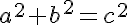### Pythagoras

Tuesday, February 24, 2009Okay, well Mr.Harbeck apparently fell, hit his head and suffered Amnesia! Don't worry he's probably okay except he thinks that we are ALIENS! Oh, there were some things in his backpack, in which he was going to teach us in class. We need to figure out what these artifacts are and how they are connected to each other. All of that, before he regains his memory!!!!!!

This is a right angle triangle(R.A.T). You know it is because it has that little square that tells you its 90°. Well the 90° is mde by two parts of the triangle( theta and beta). Those to angles make the L shape (complemetry angle). The side left over is the longest side (the hypotenuse) It is often labeled as "C". The two smaller sides are labeled as "A" or "B", it doesnt matter which one is labeled what, but usually the base is "B." The sides labeled "A" and "B" are called the legs of the triangle. REMEMBER THAT: LEGS!This is a special type of rectangle. It's a square! A square has 4 equal sides. You can tell that it has 4 equal sides because it has those lines on the sides(lines of symmetry). A square is made up of four 90° angles, one at each corner. The whole square makes 360° because of the four 90° angles. In this square, there are 2 right angle triangles. You know this because if you cut a line from one corner to the opposite corner and it'll make the 2 R.A.Ts.This is the Pythagorean Theorem. So, there was this guy named Pythagoras and he came up with the theorem to find out the length of a side of a right angle triangle. Well what the theorem means is that, if you know side "a" and side "b", then all you do is square them. From there, you will need to add them together, which will leave you with side "c" squared. Find the square root of the answer and that will give you the length of side "c".

This is a statue of Pythagoras!!! He is the great greek man that made the Pythgorean Theorem and many more. You seem interested so I will tell you more. He is known as "The Father of Mathematics and Geometry", was the first known westerner to say that the Earth revolves around the sun, he was the only westerner to be allowed in pyramids, only one to speak fluent Egyptian language, and not suprisingly he spent a lot of time in Egypt! Pythagoras liked to work with harmonics just as much as he liked math, and he died at around the age 90-100.

So now is the time to see how all these item are related to each other. I'll start with the R.A.T, it is related to all these artifacts because two right angle triangles make a square, the Pythagorean Theorem is used to find the length of a side for a R.A.T, and Pythagaros is the one who made the theorem fo rthe R.A.T.

The square is related to the right angle triangle because you can cut the square to make 2 right angle triangles. It is related to the Pythagorean Theorem, by the fact that when you add the side squared it's like and is the same as adding two squares together.

The Pythagorean Theorem is related to the R.A.T because the theorem is used to figure things out for the R.A.T. It is related to Pythagoras because he's the great man that made the theorem. It is related to the square because it is used to add the sides together.

The statue of Pythagoras is related to the R.A.T because he made the theorem for R.A.Ts. It's related to the square because it's part of the theorem he made and it'srelated to the Pythagorean theorem because he the one that made it.

Now it is time for 2 problem/question:For this question you need to find out how long the base is of the connected triangle. But to do this you need to solve for one of the triangles first. Then after you get that, you just times it by two and you'll get the length of the 2 triangles put together.This is the question: This diagram shows the game plans for a game designed by Harbeck Toys INC. The board is made up of a square and four identical right triangles. If the central square has an area of 225 square centimetres what is the perimeter of the board game?

First, find out how long each side of the square is, then that will give you side "A" and "B" for the triangles since they're both the same length. Now, like a normal question find the length of the hypotenuse ( "C" ). Once you get that you can label the triangle and add up all the sides to find the perimeter. Remember the answer won't be precise because we stop at the thousandths place and remember to make a short sentence answer!

Here are 2 videos explaining the Pythagorean Theorem: